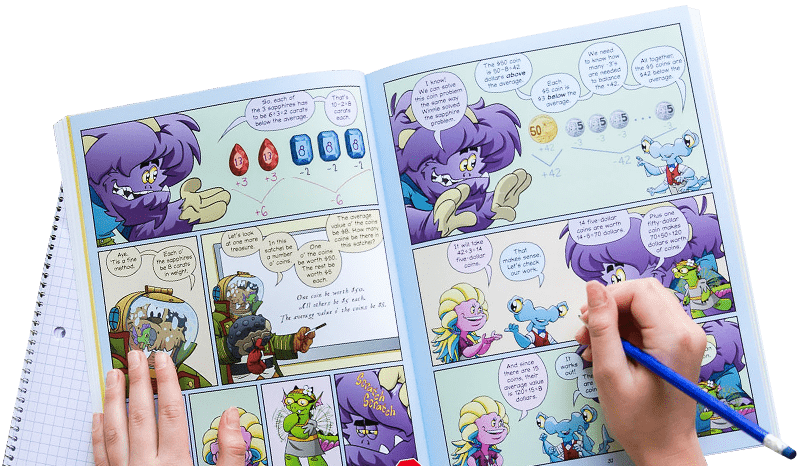All Activities U Troll Hole# Troll Hole

Look out for the troll hole as you write numbers, then add up what's left after the troll feasts.Ages: 5+Players: 2-3Time: Under 10 MinutesType: number gamesLocation: tabletopAges: 5+Players: 2-3Time: Under 10 MinutesType: number gamesLocation: tabletop

## Instructions

Draw a 19-circle hexagon. (Optional tip: trace a quarter.)

Players take turns writing numbers in the circles, starting with 1, then counting up. Each player writes in a different color. After both players have written the numbers 1-9, there will be one empty circle left: the troll hole! (Bonus: Somebody gets to draw the troll!) The troll "eats" the numbers in the circles that touch the troll hole. Cross these numbers off.

Now add the remaining numbers. This is a great chance to find pairs that add to 10. In the example above, Blue could add 7 + 3 = 10, 8 + 2 = 10, and 9 + 1 = 10 first, for a total of 30, then add the 5 + 4 = 9 remaining, for a final total of 39. Red could add 8 + 2 = 10 and 9 + 1 = 10 first, for a total of 20, then add the 5 + 4 + 7 = 16 remaining, for a total of 36. Whoever has the highest sum wins. Blue wins this game!

After a few rounds, notice that there is an easier way to discover who won the game: add the crossed-off numbers only. Whoever has the smaller sum of crossed-out numbers wins. In the game above, Blue crossed off 6, and Red crossed off 6 + 3 = 9. Since Red crossed off a higher sum, Blue wins!

Don't forget: it's Beast Academy Playground, not Beast Academy Study Hall. Change the rules, be silly, make mistakes, and try again. The Variations and Learning Notes are here for you if you want to dive deeper, but not all of them apply to learners of every age. The most important thing is to have fun.

This game is adapted from "Black Hole" by Walter Joris.

## Variations d

### More Players:

For 3 players, each player writes the numbers 1-6. Play three times, letting each player have a turn going first.

### Different Shapes:

Experiment with other shapes that can be drawn with an odd number of circles. Here are some ideas: a 25-circle diamond, a 15-circle triangle, and a 13-circle star.

Practice adding two-digit numbers. Each player starts by writing a 10 instead of a 1, and counts up from there (or pick any number to start at).

### Skip-Counting:

Rather than counting up by 1's when numbering the circles, skip-count by 2's, 3's, or whatever your child could use some practice with.

### Super Troll Hole:

Try playing with an extra hungry troll! This one eats all the numbers that are in line with the troll hole along a horizontal or diagonal line:

## Classroom Tips d

Play Troll Hole when practicing addition or subtraction skills. Modify the difficulty of the game to the level of your class (using Skip-Counting or Two-Digit Addition below).

Discussion Questions

• What other shapes can you make out of circles? Will all of these work for playing Troll Hole?
• Is there a good place to start for Player 1?
• If you're Player 2, does it matter where you start compared to Player 1?
• Is it better to have your high numbers in the corners or in the center?
• What's the next square number? (see Learning Notes)
• What's the next triangular number? (see Learning Notes)
• What other "figurate numbers" can you discover? (see Learning Notes)

• Level 2, Chapter 12: Problem Solving
• Level 4, Chapter 6: Logic

See Variations and Learning Notes for more ideas on how to adapt this activity and incorporate it into your classroom.

## Learning Notes d

### "Troll Hole" Numbers:

The 19-circle hexagon used in Troll Hole can be made larger or smaller. Give kids a chance to identify smaller hexagons hiding in the center of the game board. There is a 7-circle hexagon, and within it a 1-circle "hexagon." So, from smallest to largest, our hexagons show the numbers 1, 7, and 19. If we were to keep going, how many circles would be in the next largest hexagon? Will each of these hexagon-shaped numbers be odd? These questions can be explored by considering how each hexagon "grows" to form the next. To get from the first "hexagon" to the next, we add 6 circles for a total of 7. Next, we add 12 circles for a total of 19. This pattern continues, adding the next multiple of 6 each time to form the next hexagon. So, the next circle would be formed by adding 6 × 3 = 18 circles to the 19-circle hexagon, for a total of 19 + 18 = 37. Guide children in discovering this pattern, then challenge them to find the next few "Troll Hole" numbers.

### Figurate Numbers:

Figurate numbers can be shown by arranging objects into certain shapes. The "Troll Hole" numbers above are actually called "centered hexagonal numbers." Kids may be interested to see that other shapes also have associated sequences of numbers. Ask them to guess what the first few "triangular" or "square" numbers are.

## What do you think of this activity?

We're always looking to improve. Submit your feedback to us below.Materials
• paper
• markers (2 colors)Learning Goals
• counting
• subtraction
• strategic thinking
• pairs that add to ten
• writing numbers
• skip-countingCommon Core StandardsKeep problem solving with Beast Academy’s full math curriculum for students ages 6–13. Check out our captivating comic book series and immersive online platform.

## Bring problem-solving to your classroom

Keep your entire class engaged with a full book and online math curriculum, for students ages 6–13. 98% of teachers say they’re satisfied with Beast Academy.